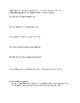# Solving equations in one-variableSubject
Grade Levels
Resource Type
Product Rating
File Type

Word Document File

Be sure that you have an application to open this file type before downloading and/or purchasing.

15 KB|2 pages
Share
Product Description
An assessment used to measure abilities when solving equations in one-variable. Students are also given written expressions to solve. It begins simple then gets more challenging. Here is the format:

Part 1:
Total of 14 problems. 6 one-step equations using each of the operations (addition, subtraction, multiplication, division, and inverse of a fraction.) 3 two-step equations. 3 two-step equations using combining like terms. 2 multi-step equations.

Part 2:
Total of 6 problems where students are given written expressions. They are to write an equation then solve. Students are given 3 one-step, 2 two-step equations, and 1 multi-step equation.

Extra Credit:
A story problem is given where students are to write an equation then solve. They equation they should produce is a one-step equation.
Total Pages
2 pages
Answer Key
Not Included
Teaching Duration
N/A
Report this ResourceSign Up